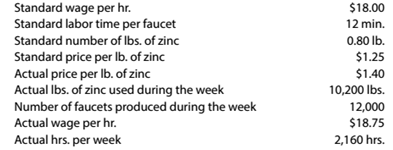Chapter 13, Problem 13.4P

Chapter
Section
Textbook Problem

Direct materials and direct labor variance analysis Faucet Industries Inc. manufactures faucets in a small manufacturing facility. The faucets are made from zinc. Faucet Industries has 60 employees. Each employee presently provides 36 hours of labor per week. Information about a production week is as follows:InstructionsDetermine (a) the standard cost per unit for direct materials and direct labor; (h) the price variance, quantity variance, and total direct materials cost variance; and (c) the rate variance, time variance, and total direct labor cost variance.

To determine

(a)

Concept Introduction:

Standard cost is an accounting system which is used by manufacturers to know the difference between actual cost and standard cost of product.

To Compute:

The standard cost per unit for direct material and direct labor.

Explanation

Details of material and labour are given in following table:

 Materials and Labours Particulars Standard Quantity Standard Price(per unit) Standard Cost Actual Quantity Actual Price(Per unit) Actual cost Material (lbs) 9,600 $1.25$12,000 10,200 $1.40$14,280 Labour (hrs) 2,400 $18$43,200 2,160 \$18
To determine

(b)

Concept Introduction:

Standard cost is an accounting system which is used by manufacturers to know the difference between actual cost and standard cost of product.

To Compute:

The price variance, quantity variance and cost variance direct material.

To determine

(c)

Concept Introduction:

Standard cost is an accounting system which is used by manufacturers to know the difference between actual cost and standard cost of product.

To Compute:

The rate variance, time variance and cost variance of direct labour.

Still sussing out bartleby?

Check out a sample textbook solution.

See a sample solution

The Solution to Your Study Problems

Bartleby provides explanations to thousands of textbook problems written by our experts, many with advanced degrees!

Get Started

Find more solutions based on key concepts